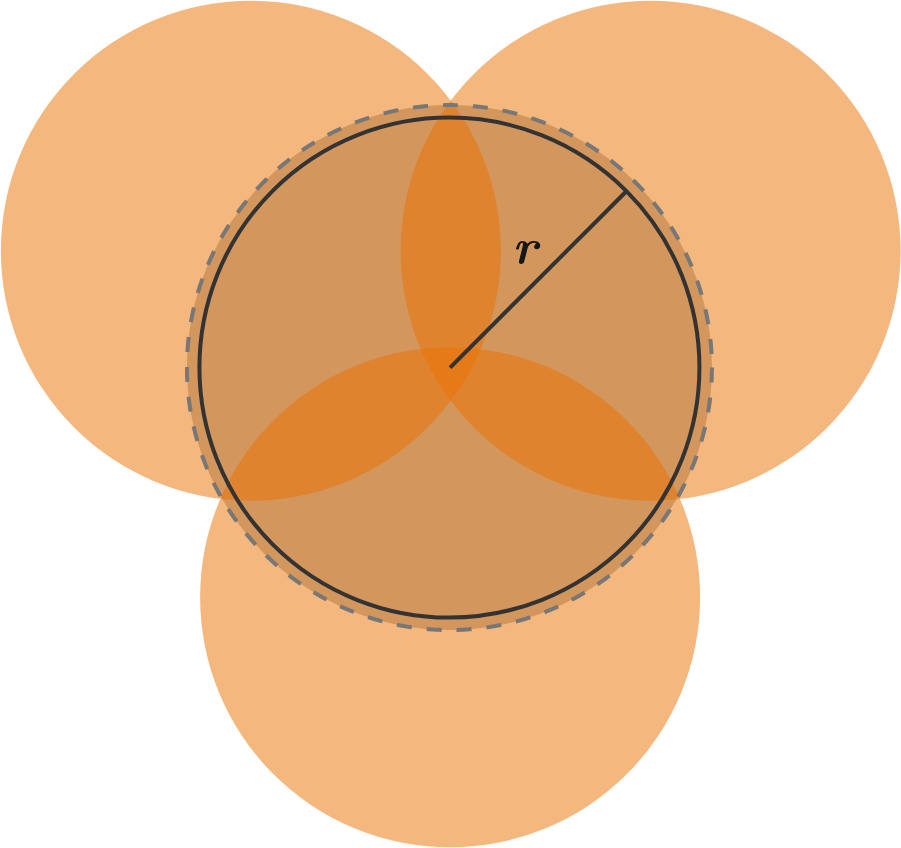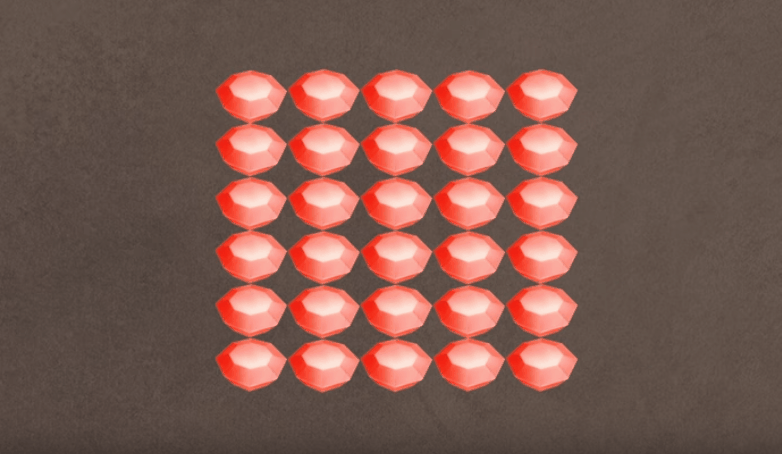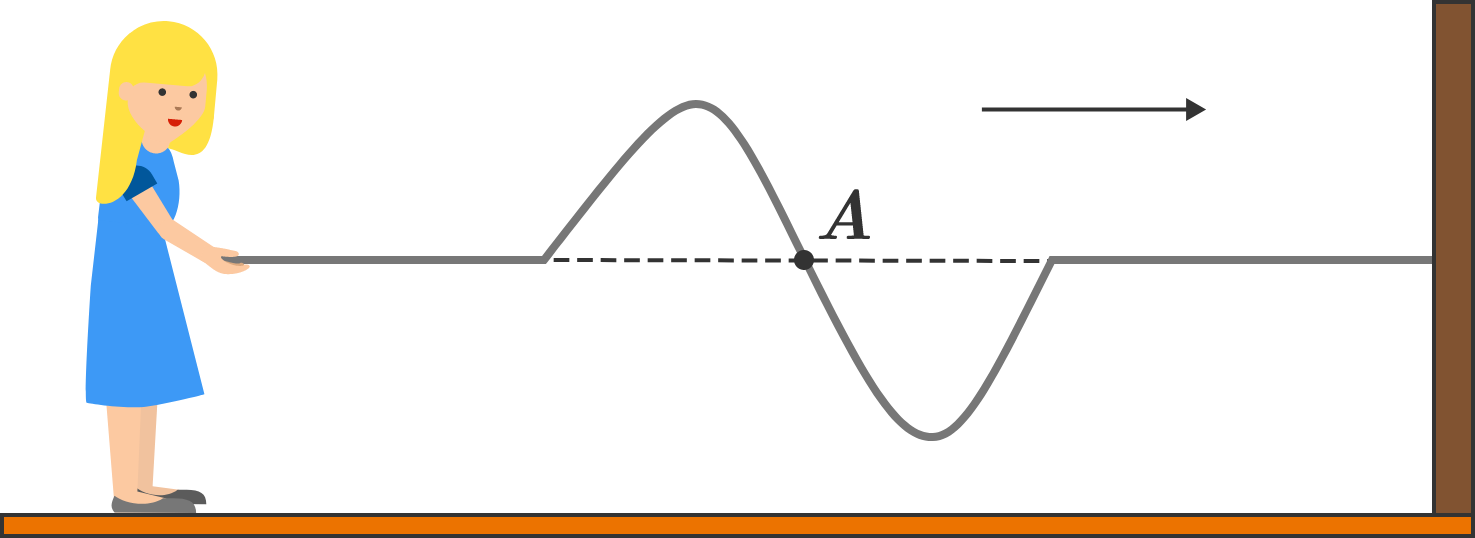# Problems of the Week

Contribute a problem

# 2018-10-22 Basic

Circles have the strange property that if you increase the size of any circle by even the tiniest amount, you need at least three circles of the original size in order to completely cover the new, slightly larger circle.The 3 overlapping orange circles have the same radius as the black circle. It takes 3 of these circles to cover the slightly larger dashed circle.

Note 1: For other shapes, rather than increasing their radius, which is generally only associated with circles, you are scaling up their shape proportionally.

Note 2: You can rotate the shapes any way you like.

A king has challenged you to a contest against a scoundrel.

You have to divide 30 rubies into 3 piles. (You can choose how many rubies go in each pile.) The scoundrel will then choose and keep 2 of those piles.

Assuming the scoundrel is greedy and wants as many rubies as possible, what is the maximum number of rubies that you can keep?Animation courtesy: TED-Ed and Artrake Studio

Bella holds the end of a rope tied to a vertical wall. Point $A$ is marked on the rope. She gives the rope a small flick to start a wave pulse, which travels towards the wall. At some instant, the rope with the wave pulse will look as shown below.

In which direction is the point $A$ moving at this instant?I have a book with regularly numbered pages, starting with 1 and increasing by 1 all the way to the last number.

Which of the following is a possible value for the total number of digits used in all the page numbers?

For example, in a book with page numbers 1 through 15, a total of 21 digits are used: $\underbrace{1+1+1+1+1+1+1+1+1}_{\stackrel{1\text{ through }9}{\text{(nine 1-digit numbers)}}}+\underbrace{2+2+2+2+2+2}_{\stackrel{10\text{ through }15}{\text{(six 2-digit numbers)}}}=21.$

• $1^5 - 1 = 0$ is divisible by 30.
• $2^5 - 2 = 30$ is divisible by 30.
• $3^5 - 3 = 240$ is divisible by 30.

True or False?

$n^{5}-n$ is divisible by 30 for all positive integers $n.$

×